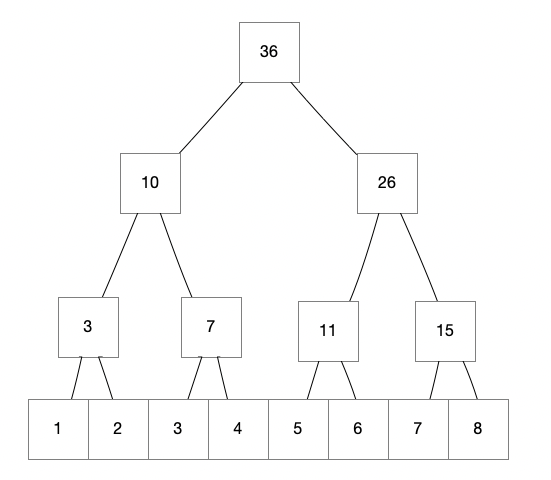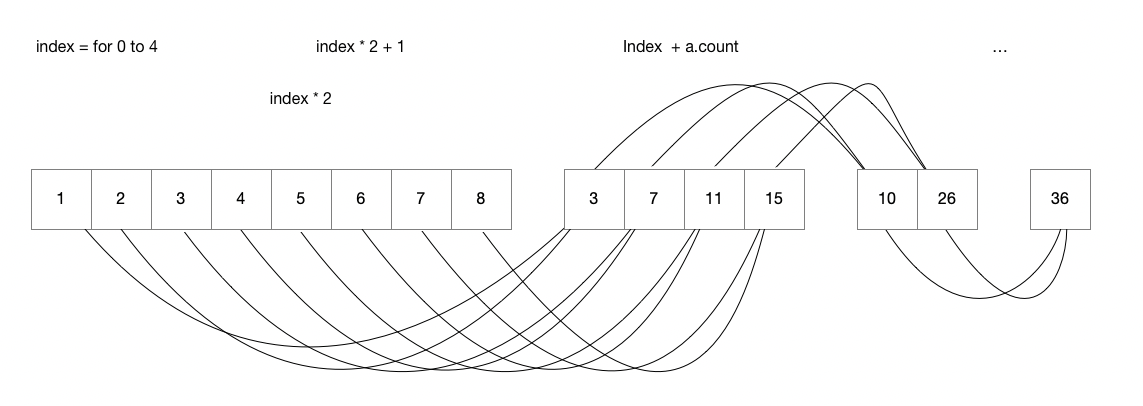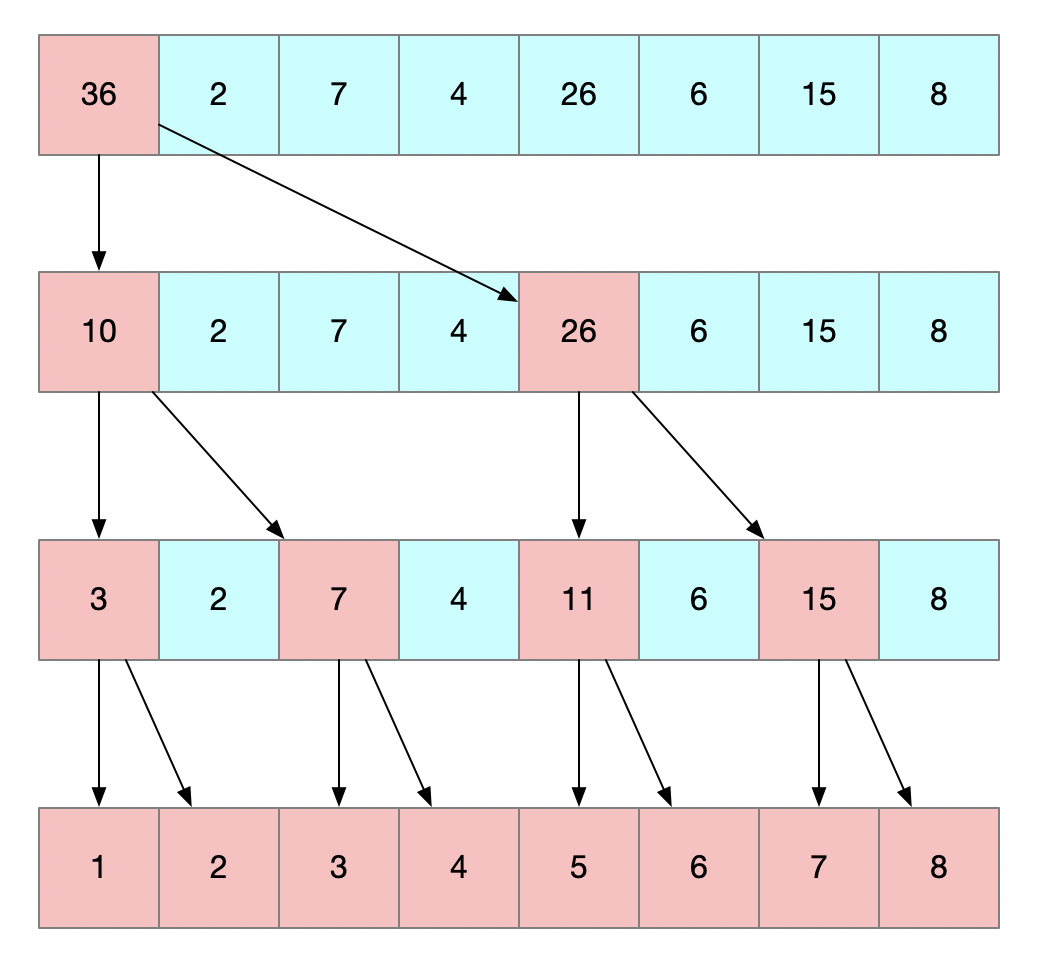Just GPU Parallel Computing…

# 0x00

No more talking, here is the original code:

This image shows how it works:But here is a problem,It’s not appropriated if you wanna use this algorithm directly.Because the resources to be calculated must be prepared in the CPU and submitted to the GPU together in metal, and returned to CPU after GPU finishes the calculation. So change the recursion to iteration might be a good idea.

# 0x01

A natural thought is to flatten the tree above and change it into an array.

eg: Declare a array :`a = [1,2,3,4,5,6,7,8]`, then we declare a new array named `b = [a,nil,nil,nil,nil,nil,nil,nil](nil means no element yet.)`, where the last three elements are the three nodes on the recursive tree, and here we go.We can use `b[index + a.count] = b[index * 2] + b[index * 2 + 1]` to calculate the value of each member.If at least one of `b[index*2],b[index*2 +1]` is nil, it means that the previous content has not been calculated yet.Use `while` to wait in a loop, the depth is `log`, so there won’t wait for more than 30 addition time.

But doesn’t work, It is because we put a while loop in a Metal shader and it does not terminate. It is fine to have a loop in Metal code, but that really only works with a fixed number of iterations. Basically, we need to rethink our logic so that it can be processed correctly on a GPU.

We need to change the way we iterate:

Here is the process diagram:In this way, the code that CPU calculated will be more complicated , because not only the `interval` need to be adjusted, the`arrayCount` need to be adjusted, but also whether the `arrayCount` is odd.Because the metal code can only handle the case where the number is even.If number is odd, the last two elements need to be manually summed and then send to the GPU for calculation.

# 0x02

for calculation 0 to 1^8, use CPU we need 32 seconds, but with GPU, the time will be shortened to 0.88 second.

Title: Swift GPU Parallel Computing

Author: Tuski

Published: 12/22/2019 - 18:01:33

Updated: 12/23/2019 - 11:35:21